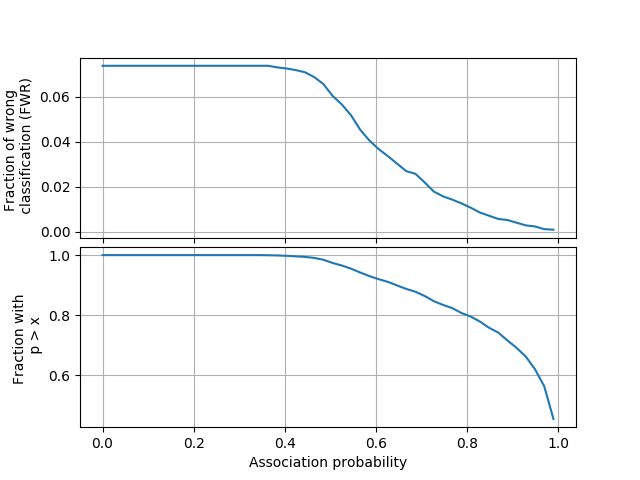Feel free to post comments in the Comments section at the end.

# Classification of Supernovae with Neural Networks

In this post I apply a Neural Network classification algorithm to a sample from a data challenge on classification, which consists of a simulated dataset of Supernovae.

There are several papers in the literature using that dataset, like this one or this one.

The full dataset can be downloaded here, and contains over 20 thousand simulated SN observed in 4 filters (g, r, i, z).

As often the case in machine learning, the data require some massaging. For each SN there are observations in multiple filters at different epochs. I interpolated these light curves on a regular time grid for each filter and then performed a continuous wavelet transform on each interpolation. The coefficients of the wavelet transform for all the filters are the features that I am going to use for the NN.

Also, in this exercise I only try to classify the SN in the three major classes: I(a), II and Ibc. I neglect the subclasses.

I will write in another post the details of the data preparation, but for the moment, I am going to use a HDF5 file which contains the dataset already prepared.

First, let’s import the packages we need:

# These settings control the level of multithreading for some operations.
# I am working on a 8 core laptop, so I use the following (adjust if needed)
import os

import pandas as pd
import numpy as np
import sklearn
from sklearn import model_selection
from sklearn import preprocessing
from sklearn import decomposition
import re

import matplotlib.pyplot as plt

%matplotlib notebook


We read the DataFrame which contains the dataset that we will use for training and cross-validation. The HDF5 file can be downloaded from here.

# Let's read back the dataframe prepared during the data preparation stage

with pd.HDFStore("store.h5") as store:

df = store['df']

# Keep only the coefficients of the wavelet transform and the sn type

def filter_function(col_name):

# The columns with the wavelet coefficients are called
# z[filter]_[number], so zg_0 is the first coefficient of
# the wavelet transform for the g filter
if re.match("z[a-z]_", col_name):

return True

# Let's also keep the sn type
if col_name=="sntype":

return True

# Apply the filter function to the column names
columns_to_keep = filter(filter_function,
df.columns.values)

# Select the columns to keep

df = df[columns_to_keep]

print("%i entries, %i features each" % (df.shape, df.shape))

20929 entries, 2561 features each


Let’s get the variable to be predicted (the SN type):

# This pops (i.e., extract) the column "sntype" from the dataframe
Y = df.pop("sntype").values


## Dimensionality reduction

We have 2560 features in our dataset (plus the SN type), which are way too many. We can apply a classic dimensionality reduction technique, the Principal Component Analysis.

However, we need to remember that we are talking about coefficients of a wavelet transform. The coefficients carry the information on the power at a particular time scale. Therefore, while usually we should rescale the features before doing a PCA, in this case we are not going to do it (otherwise we are throwing away information).

There are many alternatives to the PCA. I even tried some (LLE, Isomap…) but, despite a considerably higher computational cost, they do not bring any benefit for this dataset with respect to the PCA, so I’m going to stick with the latter.

# Perform PCA to reduce dimensionality

# WE DO NOT SCALE: wavelet power is important, if we renormalize
# we get all coefficients to the same power... if we were, we would
# have done:
# scaler = preprocessing.StandardScaler()
# df = scaler.fit_transform(df)

# Perform PCA. Since we didn't scale, we keep a fixed number of
# features (100) instead of trying to estimate the explained variance
pca = decomposition.PCA(n_components=100, svd_solver="full")
pca.fit(df.values)

# # Transform train and cross valid
X = pca.transform(df.values)


## Split train and test

We split the dataset in train and test datasets. We will not use the test dataset to train nor to optimize our algorithm’s hyperparameters, but only for a measurement of performance at the very end. We use the stratify option to keep the precentage of each class equal between training and test:

# We keep 20% of the dataset for testing
(X_t,
X_test,
Y_t,
Y_test) = sklearn.model_selection.train_test_split(X, Y,
test_size=0.20,
train_size=0.80,
stratify=Y)

print("%i items in training set, %i in test set" % (X_t.shape,
X_test.shape))

# Let's verify that we kept the percentage of each class
labels = np.unique(Y)

print("Compositions:")

for label in labels:

n_total = np.sum(Y == label) / float(Y.shape)
n_in_test = np.sum(Y_test == label) / float(Y_test.shape)
n_in_t = np.sum(Y_t == label) / float(Y_t.shape)

print("%s: %.1f (global), %.1f (training), %.1f (test)"
% (label, n_total, n_in_test, n_in_t))

16743 items in training set, 4186 in test set
Compositions:
I: 0.2 (global), 0.2 (training), 0.2 (test)
II: 0.6 (global), 0.6 (training), 0.6 (test)
Ibc: 0.1 (global), 0.1 (training), 0.1 (test)


We note how there are a lot more SN II than the other two classes, and in particular there are very few Ibc. This will explain some of the things that we are going to find later on.

## Neural Network with keras

To build our neural network we are going to use keras, by far the easiest option I have ever tried for simple experiments like this one.

If you run into problems, the easiest option to get keras and Theano up and running is by using conda and the conda-forge channel:

> conda install -c conda-forge theano keras graphviz
> pip install pydot


Let’s import what we need:

import keras
from keras.models import Sequential
from keras.layers import Dense
from keras.callbacks import EarlyStopping, ModelCheckpoint
from keras.regularizers import l2
import keras.utils

Using Theano backend.


Now let’s build our NN. Choosing the network architecture is more an art than a science, and in order to obtain good results we need to try different solutions and see which one performs better. With a slight misuse of jargon, I will call “hyperparameters” all the choices (number of layers in the network, numbers of neurons, regularization technique and parameters…). The optimization of hyperparameters is inherently dangerous if done wrong. In particular, we should never use the test set during the hyperparameter optimization. Instead, usually we would use a cross-validation dataset, which is a split taken from the training set (not the test set), for testing our choices and optimize the hyperparameters and then run the result on the test set to measure performances.

However, since the dataset in this case is not very large, I prefer to use (stratified) k-fold cross-validation. It works like this: the training dataset is divided in $k_i$ equally-sized splits, with $i=0..k-1$. For each one of $k$ iterations, the $k_i$ split is used as cross-validation dataset and the union of the other k-1 splits as traning set, and the performance of the network evaluated. At the end, the performance is averaged over the k iterations. The target of the optimization of the hyperparameters is the averaged performance, so that the risk of overfitting a particular cross validation dataset is reduced.

I already did this tiresome procedure and found a good model. However, the good news is that results don’t vary too much for a wide range of choices. After my experiments, I get nice results with 2 hidden layers of 70 units each with a rectifier activation function (plus of course the output layer, in which I use the softmax activation so I can interpret the output as a probability of association). I also use L2 regularization to avoid the disproportionate weighting of some features (see here for a nice introduction). There is probably space for some more hyperparameters optimization, but this is quite good already.

If you want to experiment, this is the setup. First we define a factory class that can be used to setup and get a trained network (and other things). The network is defined inside the _get_network method, so if you want to try other architerctures/hyper-parameters you need to change things in there:

# I use a factory class to build the classifier because I am going to use
# the same network many times with different datasets

class ClassifierFactory(object):

def __init__(self, n_dim, n_classes, l2_lambda=0.01):

self._n_dim = int(n_dim)
self._n_classes = int(n_classes)
self._l2_lambda = float(l2_lambda)

# On initialization the sets are empty
self._X_t = None
self._Y_t = None
self._X_cv = None
self._Y_cv = None

def _get_network(self):

# Setup NN from scratch

classifier = Sequential()

# Hidden layer 1 (the input one is added automatically)
units=70,
kernel_initializer='uniform',
activation='relu',
kernel_regularizer=l2(self._l2_lambda),
name="Layer 1"))

# Hidden layer 2
kernel_initializer='uniform',
activation='relu',
kernel_regularizer=l2(self._l2_lambda),
name="Layer 2"))

# Output layer
kernel_initializer='uniform',
activation='softmax',
name="Output"))

# Compile network
loss='categorical_crossentropy',
metrics=['accuracy'])

return classifier

def set_training_set(self, X_t, Y_t):
"""
Store the training set
"""
self._X_t = X_t
self._Y_t = Y_t

def set_cross_validation_set(self, X_cv, Y_cv):
"""
Store the cross validation set (use None and None to remove
a previously stored cross-validation dataset)
"""
self._X_cv = X_cv
self._Y_cv = Y_cv

@staticmethod
def _hot_encoder(this_Y):
"""
Utility method to encode a label column using one hot encoder
see https://goo.gl/d6PPpr for an introduction
"""
label_encoder = preprocessing.LabelEncoder()
integer_encoded = label_encoder.fit_transform(this_Y)

# binary encode
onehot_encoder = preprocessing.OneHotEncoder(sparse=False)
integer_encoded = integer_encoded.reshape(len(integer_encoded), 1)
onehot_encoded = onehot_encoder.fit_transform(integer_encoded)

return onehot_encoded, label_encoder

def train_and_get(self, use_weights=False, quiet=False, n_epochs=None):
"""
Build, train and return the network, the training history and the
label encoder/decoder. Parameters:

:param use_weights: whether to use weights to balance unbalanced
classes
:param quiet: suppress most of the screen output
:param n_epochs: if None (default) the training is stopped with
early stopping when the validation accuracy stops
improving. If an integer value is provided, instead,
exactly n_epochs will be used for training.
"""

# Get a new network
classifier = self._get_network()

# Encode labels

onehot_encoded, label_encoder = self._hot_encoder(self._Y_t)

if self._Y_cv is not None:

# Encode cross-validation labels

onehot_encoded_cv, label_encoder_cv = self._hot_encoder(self._Y_cv)

if use_weights:

# We try to compensate for the unbalance between the classes in
# the training sample by using the weights in the training

if not quiet:

print("Using these weights: ")

weights = np.zeros(self._Y_t.shape, dtype=float)

n_elements = self._Y_t.shape

for label in labels:

n = (self._Y_t == label).sum()

this_weight = 1 - (n / float(n_elements))

if not quiet:

print("%s: %.2f" % (label, this_weight))

idx = (self._Y_t == label)
weights[idx] = this_weight

else:

weights = None

callbacks = []

# Setup the training

if n_epochs is None:

if not quiet:

print("Using early stopping")

# To avoid overtraining (aka memorization), we use early
# stopping, i.e., keras stops the training when the accuracy
# on the validation dataset stops improving.

n_epochs = 1000 # We'll never reach 1000 epochs because
# of early stopping

early_stopping = EarlyStopping(monitor='val_acc',
patience=30,
verbose=0)

callbacks.append(early_stopping)

# We use checkpointing, i.e., we keep track of the iteration with
# the best accuracy and save it to a file.  Since there is some
# randomness involved in the training, the val_acc could be at
# its maximum not in the very last epoch.

check_point = ModelCheckpoint("best_weights",
monitor='val_acc',
verbose=0,
save_best_only=True,
save_weights_only=True,
mode='max')

callbacks.append(check_point)

if self._X_cv is not None and self._Y_cv is not None:

# We have a cross-validation dataset, let's use it
validation_data=(self._X_cv, onehot_encoded_cv)

else:

print("No cross-validation used")

# We do not use any cross-validation
assert n_epochs is not None, ("You cannot use early stopping "
"at the same time as a fixed "
"number of epochs")

validation_data=None

history = classifier.fit(self._X_t, onehot_encoded,
batch_size=self._X_t.shape,
epochs=n_epochs,
validation_data=validation_data,
verbose=0,
shuffle=False,
callbacks=callbacks,
sample_weight=weights)

# Now load the weights which gave the best validation accuracy

return classifier, history, label_encoder


### Train

Before repeating the training/evaluation $k$ times, let’s do it manually once first to make sure everything works as expected. We can get the classifier and train it using the factory we have built above. But first we need a cross-validation sample (during k-fold cross-validation this will be one of the $k$ splits):

# Take out a cross-validation dataset (20 % of the training set)
(X_tstar,
X_cv,
Y_tstar,
Y_cv) = model_selection.train_test_split(X_t, Y_t,
test_size=0.20,
train_size=0.80,
stratify=Y_t)

print("%i items in training set, %i in test set" % (X_tstar.shape,
X_cv.shape))

# Instance the factory
factory = ClassifierFactory(n_dim=X_t.shape, n_classes=3)

# Set training and cross-validation sets
factory.set_training_set(X_tstar, Y_tstar)
factory.set_cross_validation_set(X_cv, Y_cv)

# Get the classifier, the history and the label encoder
classifier, hist, label_encoder = factory.train_and_get()

13394 items in training set, 3349 in test set
Using early stopping


Let’s also have a look at our network. The plot is not super nice (what’s up with all those None?) but it gets the idea across:

from IPython.display import Image
from keras.utils.vis_utils import model_to_dot

dot = model_to_dot(classifier, show_layer_names=True, show_shapes=True,
rankdir='TB')

Image(dot.create_png())Now that the training is done, we can see how the training has progressed with the epochs:

fig, sub = plt.subplots()

_ = plt.plot(hist.history['acc'], '.', label='Training accuracy')
_ = plt.plot(hist.history['val_acc'], '.', label='Validation accuracy')

_ = plt.plot(hist.history['loss'], '.', label='Training loss')
_ = plt.plot(hist.history['val_loss'], '.', label='Validation loss')

plt.xlabel("Epoch")

plt.ylim([0, 2])

_ = plt.legend()

<IPython.core.display.Javascript object>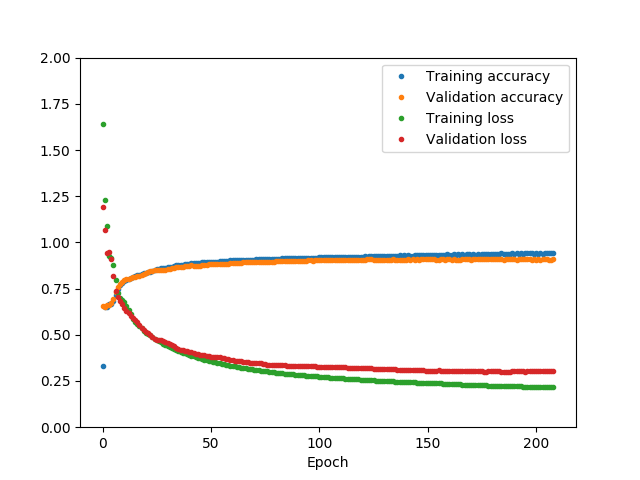We can see that towards the end, while the training loss keep decreasing, the validation loss does not, i.e., we are starting to “memorize” the training dataset instead of improving our generalization power. This is where the early stopping we have implemented kicks in and stops the traning. In the final epoch the training and validation accuracy are similar. Also the training loss is smaller than the validation loss, but not too much. These factors indicates that we have reached a good compromise and we are likely not overfitting nor underfitting too much.

## K-fold stratified cross-validation

Here we perform the k-fold stratified cross-validation, as explained above. It is actually pretty easy to perform (but it takes a few minutes):

from sklearn.model_selection import StratifiedKFold

# Using k=10 we keep every time 10 percent of the sample as cross-validation
k = 10

skfold = StratifiedKFold(n_splits=k, shuffle=True)

acc = []
loss = []
val_acc = []
val_loss = []
stop_epoch = []

for i, (train_idx, cv_idx) in enumerate(skfold.split(X, Y)):

# Get the train/cross-validation datasets

Xx_t, Yy_t = X[train_idx], Y[train_idx]
Xx_cv, Yy_cv = X[cv_idx], Y[cv_idx]

# Get a classifier trained on this train dataset and cross-validated
# on this cross-validation dataset
factory.set_training_set(Xx_t, Yy_t)
factory.set_cross_validation_set(Xx_cv, Yy_cv)
# Let's use weighting, so we compensate a little for the lack of many
# Ibc supernovae in the training set
classifier, hist, label_encoder = factory.train_and_get(quiet=True, use_weights=True)

# Record performance metrics
acc.append(hist.history['acc'][-1])
loss.append(hist.history['loss'][-1])
val_acc.append(hist.history['val_acc'][-1])
val_loss.append(hist.history['val_loss'][-1])

# Let's also keep track of when the early stopping actually
# stopped the training
stop_epoch.append(len(hist.history['val_loss']))

print("%i done" % i)

0 done
1 done
2 done
3 done
4 done
5 done
6 done
7 done
8 done
9 done


Let’s see how the accuracy varies across the $k$ iterations:

fig, sub =plt.subplots()

_ = plt.plot(range(k), acc, label='Training accuracy', alpha=0.2, marker='o')

_ = plt.plot(range(k), val_acc, label='Validation accuracy', marker='o')

plt.xlabel("k")

_ = plt.legend(loc=0)

<IPython.core.display.Javascript object>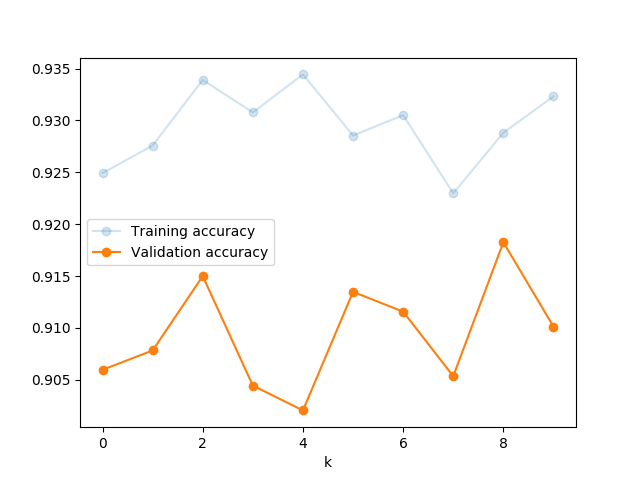There is not too much variation among the repetitions. We can see that the validation accuracy is between 90 and 93 percent, and the training accuracy is similar.

If you want to try improving you should repeat the previous step up to here. DO NOT use the test set to improve your network. Only when you have finished optimizing the configuration, you can proceed with testing on the test set.

Now let’s see when the early stopping actually stopped the training:

fig, sub = plt.subplots()
sub.set_xlabel("k")
sub.set_ylabel("Last iteration")

_ = sub.plot(range(len(stop_epoch)), stop_epoch, '.')

<IPython.core.display.Javascript object>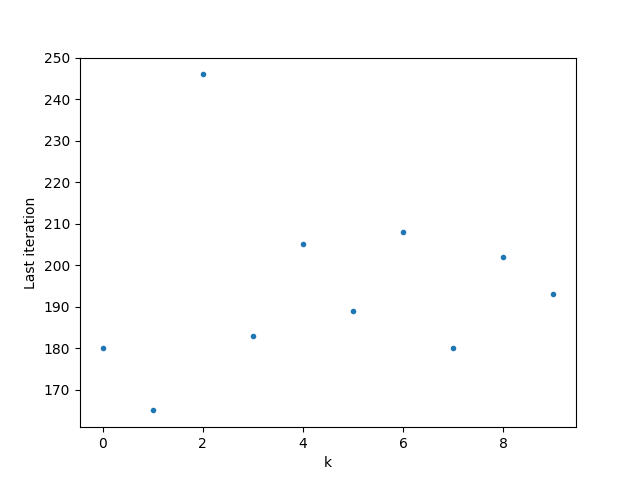Keeping in mind that we will now have 10% more samples, I think a number of epochs of 300 should be enough to train the dataset and avoid overtraining. Let’s proceed with the training on the entire training dataset:

factory.set_training_set(X_t, Y_t)

# We do not use a cross-validation set here
factory.set_cross_validation_set(None, None)

classifier, hist, label_encoder = factory.train_and_get(n_epochs=400, quiet=True, use_weights=True)

No cross-validation used

/home/giacomov/miniconda2/envs/tensorflow/lib/python2.7/site-packages/keras/callbacks.py:402: RuntimeWarning: Can save best model only with val_acc available, skipping.
'skipping.' % (self.monitor), RuntimeWarning)


### Measure performance

Now that we have trained the classifier, we can evaluate its performance on the test set that we did not use for training:

# Make prediction on the test set

pred_hc = classifier.predict(X_test)

pred = label_encoder.inverse_transform(np.argmax(pred_hc, axis=1))

print("Accuracy on test sample: %.2f" % ((pred == Y_test).sum() / float(X_test.shape)))

Accuracy on test sample: 0.93


We have reached an accuracy of around 93 percent on the test dataset, not bad at all. Of course, this number can change a bit depending on the random choice of the test set that occurred at the beginning (try by re-running the entire notebook…). However, it won’t change much, a few percent point at most.

Our classificator also can give the probability of association with each class. The prediction of the classifier for one sample is indeed just the class with the highest association probability for that sample. It is interesting to build a ROC curve considering each class separately as positive (and the others combined as negative). This gives an idea of how good is the classifier in classifying each class separately:

from sklearn.metrics import roc_curve, auc

fpr = {}
tpr = {}
roc_auc = {}

labels = np.unique(Y_test)

for i, label in enumerate(labels):

# Get the probability of association for each individual in the cross-validation dataset
# (NOTE: this is one probability for each class, for each individual)
pred_hc_prob = classifier.predict_proba(X_test, verbose=0)

# Get the true binary classification
# (1 if the true class is "label", 0 if not)
y_true = (Y_test == label)

# Get the classifier probability that each individual belong to
# the class "label"
y_score = pred_hc_prob[:, i]

# Compute the ROC curve and the Area under the curve (AUC)

fpr[label], tpr[label], _ = roc_curve(y_true, y_score)

roc_auc[label] = auc(fpr[label], tpr[label])

# Now plot them

fig, sub = plt.subplots()

for label in labels:

_ = sub.plot(fpr[label], tpr[label], label='%s (area = %0.2f)' % (label, roc_auc[label]))

_ = sub.plot([0, 1], [0, 1], color='navy', lw=2, linestyle='--')

sub.set_xlabel('False positive rate')
sub.set_ylabel('True positive rate')
sub.set_title('ROC curve')

_ = plt.legend(loc=0)

<IPython.core.display.Javascript object>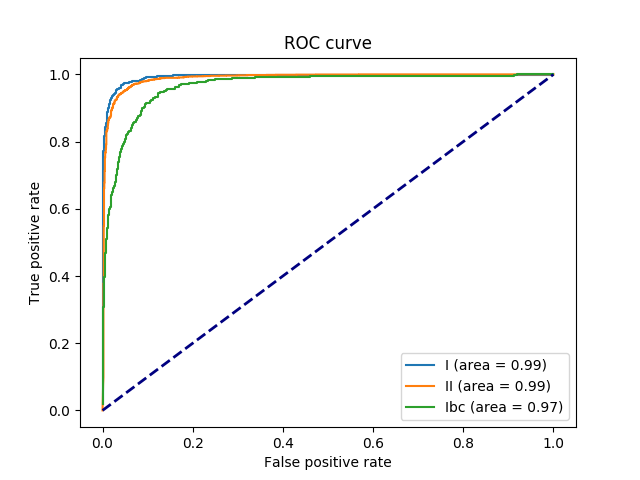The classifier generally behaves very well for each class, but it appears that classifying supernovae Ibc is a little more difficult than the other two classes. This might be related to the fact that there are very few Ibc in the training sample, and so the classifier is optimized more for the other two classes. Applying a weighting during training makes a small difference (you can try removing it if you want and see what happens). This is still a very high performance, but if an improvement is needed we probably need more Ibc samples.

There is also another way of looking at the same problem “globally”, i.e., without dividing the classes. Since we have the probability of association, we can use it to clean our sample. Without any further cut, our NN assign a class to an individual SN as the one with the highest probability at the end of the tree. However, if an individual is assigned a label such as “Type Ia” with only say 51% probability, we expect that this classification is less reliable than another individual for which the probability is 90%. It is interesting to study how much the classification mistakes decrease as the probability of association increases. At the same time, it is interesting to study the fraction of individuals above a certain probability of association. This can be done as follows:

# Get the probability of association for each individual in the cross-validation dataset
# (NOTE: this is one probability for each class, for each individual)
pred_hc_prob = classifier.predict_proba(X_test, verbose=0)

# For each individual, get the max probability
pred_hc_prob_mx = np.max(pred_hc_prob, axis=1)

# Let's make a grid of 50 probabilities
prob_cuts = np.linspace(0, 0.99, 50)

# The fraction of individuals with wrong classification is called
# Family-Wise error Rate (FWR)
fwr = []

# This will contain the fraction of individuals with p > p*
completeness = []

# Number of individuals
n = X_test.shape

for p in prob_cuts:

# Apply the cut
cut_idx = (pred_hc_prob_mx >= p)

# Select the wrong associations within the individuals kept after
# the previous cut
wrong_idx = (pred != Y_test) & cut_idx

# Compute the FWR

fwr.append(np.sum(wrong_idx) / float(n))

# Compute the completeness

completeness.append(np.sum(cut_idx) / float(n))

fig, subs = plt.subplots(2, 1, sharex=True)

_ = subs.plot(prob_cuts, fwr, label='Fraction of wrong classification (FWR)')
_ = subs.set_ylabel("Fraction of wrong \n classification (FWR)")
_ = subs.grid()

_ = subs.plot(prob_cuts, completeness, label='Completeness')
_ = subs.set_xlabel("Association probability")
_ = subs.set_ylabel("Fraction with \n p > x")
_ = subs.grid()


<IPython.core.display.Javascript object>